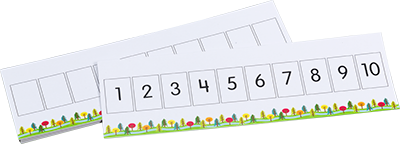"With current math standards' emphasis on number lines as a visual math model, number lines are being used in nearly all primary grades classrooms for learning such things as counting and early operations. However, research has shown that number lines are conceptually too difficult for young children to understand and instead we should be using number paths, at least until second grade (Fuson, et. al., 2009).

We caught up with Margaret McGinty, educator and creator of the new Number Path Pocket Chart, and asked her to elaborate on why number paths are a better choice than number lines in kindergarten and first grade.

## Q: How are number paths different from number lines?

A: A number path is a counting model. Numbers are represented by rectangles and each rectangle can be counted. A number line is a length model, like a ruler. Each number is represented by its length from zero.

## Q: What makes a number line more difficult to use?

A: With a number line, children have to count the length units and not the numbers. Number lines make it difficult for young children to see the units being counted. Sometimes when young children use a number line, they start their count with zero and then are off by one. Some count the spaces between numbers and when they end their count, their finger is between two numbers and they do not know which number to use.

When students start using a number line before they are ready, they are seeing spaces and lines rather than concrete quantities. In this sense, number lines take the actual quantity of a number out of student understanding.## Q: In what ways is a number path an improvement over a number line?

A: Counting errors, such as those described above, do not occur on a number path. On a number path the units being counted are simple to recognize. Each rectangle, or box, houses its own number. This supports the one-to-one correspondence that early mathematicians need to have and master. As children count along the number path, they actually count one whole box as they move from one number to the next, say from 4 to 5. In addition the boxes are large enough for young children to touch each box and see that one box equals one number.

Students can move along the number path adding by 1's or 2's and actually seeing where those addends come from. Similar to a game of hopscotch, this is a concrete way for students to count and see relationships and patterns among numbers.

## Q: But if students are expected to use a number line in second grade, shouldn't they start with that model from the beginning?

A: When young children are still making sense of numbers, we don't want to use models that confuse them. We want models that help build their understanding. The inherent simplicity of the number path satisfies the rule that a model should help build understanding and fluency with ease.

## Q: Can you give us some examples of how students are using Number Paths?

A: Sure. Here are a few.

1. Kids play a roll-and-compare game by each rolling a die and placing a marker on the number rolled. Whoever has the greater or lesser number, or even or odd number, or whatever is being compared, gets the point. There are many possibilities for comparing numbers in this format.
2. Kids practice addition by rolling a die, putting a counter on the number rolled, and then rolling again and using the number path to add that number to get the sum. The number path is a great tool for making combinations of 10.
3. Kids use the number path to touch each number as they count out loud or count around a circle for choral counting.

## Q: Tell us about the Number Path Pocket Chart. Why did you create it?

A: The chart allows teachers to create number paths with cards showing multiple representations of the numbers 1 to 10: numerals, number words, dot configurations, ten-frames, and hands. The pocket chart is ideal for developing number sense, subitizing, and making tens."

### Number Path Sample Activities

Here are some simple but highly instructive activities you can try with your students in Kindergarten through Grade 2, as you familiarize them with working with number paths.

Sample Activities